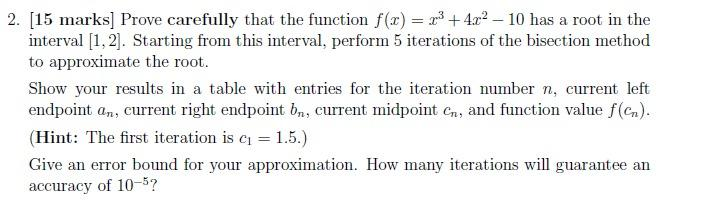# Question 2. (15 marks] Prove carefully that the function f(x) = x3 + 4x2 - 10 has a root in the interval [1,2]. Starting from this interval, perform 5 iterations of the bisection method to approximate the root. Show your results in a table with entries for the iteration number n, current left endpoint an, current right endpoint bn, current midpoint Cr, and function value f(cn). (Hint: The first iteration is c = 1.5.) Give an error bound for your approximation. How many iterations will guarantee an accuracy of 10-5?